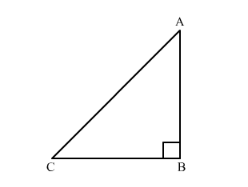# An aeroplane leaves an airport and flies due north at a speed of 1000 km/hr.

Question:

An aeroplane leaves an airport and flies due north at a speed of $1000 \mathrm{~km} / \mathrm{hr}$. At the same time, another aeroplane leaves the same airport and flies due west at a speed of $1200 \mathrm{~km} / \mathrm{hr}$. How far apart will be the two planes after $1 \frac{1}{2}$ hours?

Solution:

Let us draw the figure first.An aeroplane which flies due north at a speed of $1000 \mathrm{~km} / \mathrm{hr}$ covers the distance $\mathrm{AB}$ after $1 \frac{1}{2} \mathrm{hr}$ and another aeorplane that flies due west at the speed of $1200 \mathrm{~km} / \mathrm{hr}$ covers the distance $\mathrm{BC}$ after $1 \frac{1}{2} \mathrm{hr}$.

We know that speed $=\frac{\text { distance }}{\text { time }}$

$\therefore$ distance $=$ speed $\times$ time

Let us calculate AB first as shown below,

$A B=1000 \times 1.5$

$\therefore A B=1500 \mathrm{~km}$

Similarly we can calculate BC.

$B C=1200 \times 1.5$

$\therefore B C=1800 \mathrm{~km}$

Now we have find AC. To find AC we will use Pythagoras theorem,

$A C^{2}=A B^{2}+B C^{2}$

$\therefore A C^{2}=(1500)^{2}+(1800)^{2}$

$\therefore A C^{2}=2250000+3240000$

$\therefore A C^{2}=5490000$

Taking square root we get,

$A C=2343.07$

Therefore, after $1 \frac{1}{2}$ hrs the aeroplanes will be approximately $2343 \mathrm{~km}$ far apart.